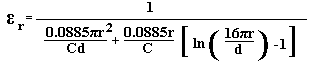# Accurate Method for Measuring Relative Dielectric Constant of a PCB Using a Vector Network Analyser and parallel plates.

1) Introduction

Relative dielectric constant is traditionally measured at 1 MHz on a sheet of PCB material and this produces erroneous results because skin effect and fringing are not taken into account. By using the method shown here, these errors will be removed and a more accurate result obtained.

2) Prepare a Sample

Prepare a sample PCB which shall have a circular sample on the top and a concentric mirrored ground on the bottom. The sample shall be surrounded by at least 1 cm of bare dielectric. It may assist to put small circles at the corners of the PCB in order to define the boundaries. The sample shall have one fine hole at the centre, just large enough to insert the centre conductor of a piece of semi rigid coax. The size of the sample can be selected by using the PCB Sample Size Calculator for relative dielectric constant measurements.

3) Connect Sample

Select a short piece of semi rigid (5 cm is adequate) with a suitable connector at one end. Short the open end and then connect the semi rigid to the VNA, which should be ready calibrated with a sweep of about 3 GHz. Using the Smith Chart Format, perform a port extension with the VNA, which should produce a "dot" at the left hand side of a Smith Chart. Disconnect the semi rigid from the VNA and then remove the short. Make a very small countersink at the centre of the PCB sample just large enough to ensure that the semi rigid inner does not short to the bottom plane. Insert the semi rigid into the sample and solder at both sides. Reconnect to the VNA.

4) Ensure the Sample Size is Adequate

Using the Smith Chart Format on the VNA ensure that the trace starts at the right hand side (00) at the minimum frequency and sweeps clockwise. The trace should be very close to the perimeter of the chart all of the way to + 1800. If the trace does not reach + 1800, then the sweep of the VNA is inadequate or the sample size is too small.

Set the marker for exactly  -900. This can be achieved by selecting the Polar Format or Phase , setting the marker for -900 and leaving the marker at this frequency, select the Smith Chart Format again. The marker should still be at -900 point and R -j Ohms should be indicated. The -j reading shall be 49.8Ω to 50Ω for a Zo of 50Ω i.e. almost a pure reactance. If the capacitive reactance is less than this, the sample size is probably too large in relation to the thickness of copper.

5) Taking the reading

The VNA should indicate the capacitance at -900, but if you are using a VNA without this facility the capacitance can be calculate from the reactance and the frequency using:-

C = 1 / (2πfXc)

6) Compute the Relative Dielectric Constant

The result can be calculated using the RDC Calculator or by the following formula.7) Wrong Size Sample

If the sample size was too large or too small, it is most likely that the original data used to calculate the sample size was too far from the actual characteristics. If you suspect that this is the case, repeat the exercise using the εr that was achieved in the result above. It should be in the right ball park area!

If the thickness of the board is in doubt, then measure it with a micrometer at a point where there is no copper on either side. The measurement will need to be made very carefully with a micrometer. A vernier is not accurate enough. This is probably the most critical part of the whole process!

If the copper thickness is in doubt, then it is possible to plot a curve and find the frequency at which the capacitance value peaks, from this the copper thickness can be calculated. Refer to the RDC Article for this. Alternatively the copper size can be calculated from the thickness of the board with copper minus the thickness of the board without copper, measuring with a micrometer.

Return to Technical Articles William J Highton 25/10/2004